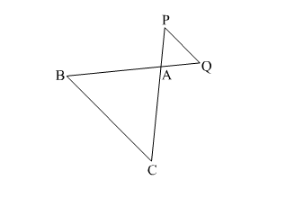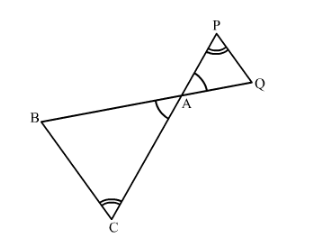# In the given figure, $riangle mathrm{ACB} sim riangle mathrm{APQ}$.

Question:

In the given figure, $\triangle \mathrm{ACB} \sim \triangle \mathrm{APQ}$. If $\mathrm{BC}=10 \mathrm{~cm}, \mathrm{PQ}=5 \mathrm{~cm}, \mathrm{BA}=6.5 \mathrm{~cm}$ and $\mathrm{AP}=2.8 \mathrm{~cm}$, find $\mathrm{CA}$ and $\mathrm{AQ}$. Also, find the area $(\triangle \mathrm{ACB}):$ area $(\triangle \mathrm{APQ})$.Solution:

Given: ΔACB is similar to ΔAPQ.

BC = 10 cm, PQ = 5cm, BA = 6.5cm and AP = 2.8 cm

TO FIND:

(1) CA and AQ

(2) Area of ΔACB : Area of ΔAPQ

(1) It is given that ΔACB ~ ΔAPQ.We know that for any two similar triangles the sides are proportional. Hence

ABAQ=BCPQ=ACAP

$\frac{\mathrm{AB}}{\mathrm{AQ}}=\frac{\mathrm{BC}}{\mathrm{PQ}}$

$\frac{6.5}{\mathrm{AQ}}=\frac{10}{5}$

$\mathrm{AQ}=3.25 \mathrm{~cm}$

Similarly,

$\frac{\mathrm{BC}}{\mathrm{PQ}}=\frac{\mathrm{CA}}{\mathrm{AP}}$

$\frac{\mathrm{CA}}{2.8}=\frac{10}{5}$

$\mathrm{CA}=5.6 \mathrm{~cm}$

(2) We know that the ratio of areas of two similar triangles is equal to the ratio of squares of their corresponding sides.

ar∆ACQar∆APQ=BCPQ2=1052=212=41